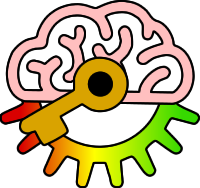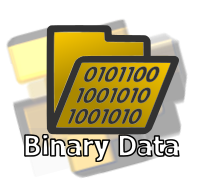# Y8

4j: Convert a decimal number to binary and vice versa.

Keywords:Test your self on these keywords and definitions using the games below or
KeywordDefinition
binarya base 2 number system (using digits 0 and 1) where each digit is worth 2 times as much as the digit to its right
decimala base 10 number system (using digits 0-9) where each digit is worth 10 times as much as the digit to its right
denaryanother name for decimal
unsigneda number that can only greater than or equal to zero
Keyword games:
Resources: